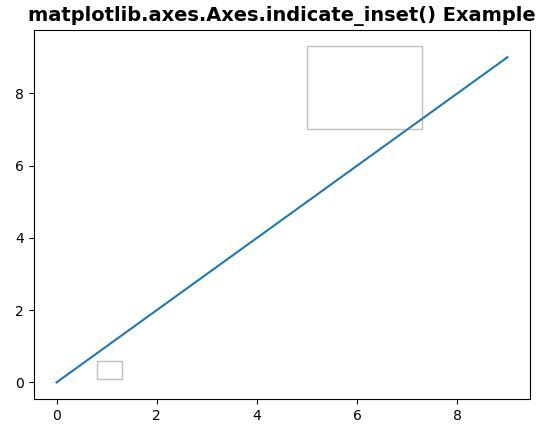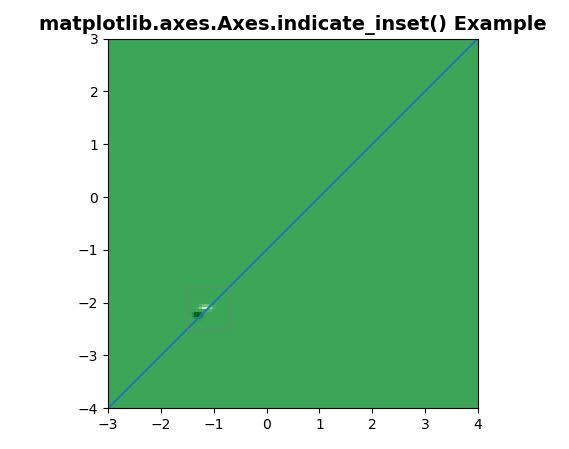# Matplotlib.axes.Axes.indicate_inset() in Python

• Difficulty Level : Expert
• Last Updated : 19 Apr, 2020

Matplotlib is a library in Python and it is numerical – mathematical extension for NumPy library. The Axes Class contains most of the figure elements: Axis, Tick, Line2D, Text, Polygon, etc., and sets the coordinate system. And the instances of Axes supports callbacks through a callbacks attribute.

## matplotlib.axes.Axes.indicate_inset() Function

Attention geek! Strengthen your foundations with the Python Programming Foundation Course and learn the basics.

To begin with, your interview preparations Enhance your Data Structures concepts with the Python DS Course. And to begin with your Machine Learning Journey, join the Machine Learning - Basic Level Course

The Axes.indicate_inset() function in axes module of matplotlib library is also used to add an inset indicator to the axes.

Syntax: Axes.indicate_inset(self, bounds, inset_ax=None, *, transform=None, facecolor=’none’, edgecolor=’0.5′, alpha=0.5, zorder=4.99, **kwargs)

Parameters: This method accept the following parameters that are described below:

• bounds: This parameter is the Lower-left corner of rectangle to be marked and its width and height.[x0, y0, width, height]
• transform: This parameter is the units of rect are in axes-relative coordinates.
• zorder: This parameter contains the number and its default value is 5.
• inset_ax: This parameter ia an optional inset axes to draw connecting lines to.
• facecolor: This parameter is used to insert the facecolor of the rectangle.
• edgecolor: This parameter is the color of the rectangle and color of the connecting lines.
• alpha: This parameter represents the transparency of the rectangle and connector lines.

Returns: This method returns the the following:

• rectangle_patch : This return the indicator frame.
• connector_lines: This return the four connector lines connecting to (lower_left, upper_left, lower_right upper_right) corners of inset_ax.

Note: This function works in Matplotlib version >= 3.0

Below examples illustrate the matplotlib.axes.Axes.indicate_inset() function in matplotlib.axes:

Example 1:

 `# Implementation of matplotlib function``import` `matplotlib.pyplot as plt``   ` `fig, ax ``=` `plt.subplots()``ax.plot(``range``(``10``))``axin1 ``=` `ax.indicate_inset([``0.8``, ``0.1``, ``0.5``, ``0.5``])``axin2 ``=` `ax.indicate_inset(``        ``[``5``, ``7``, ``2.3``, ``2.3``], transform ``=` `ax.transData)``ax.set_title(``'matplotlib.axes.Axes.indicate_inset() Example'``,``             ``fontsize ``=` `14``, fontweight ``=``'bold'``)``plt.show()`

Output:Example 2:

 `# Implementation of matplotlib function``import` `matplotlib.pyplot as plt``import` `numpy as np``  ` `def` `geeks():``    ``from` `matplotlib.cbook ``import` `get_sample_data``    ``import` `numpy as np``    ``f ``=` `get_sample_data(``"axes_grid/bivariate_normal.npy"``,``                        ``asfileobj ``=` `False``)`` ` `    ``z ``=` `np.load(f)``    ``return` `z, (``-``3``, ``4``, ``-``4``, ``3``)``  ` `fig, ax ``=` `plt.subplots()``ax.plot(``range``(``-``3``, ``5``), ``range``(``-``4``, ``4``))``X, extent ``=` `geeks()``Z2 ``=` `np.zeros([``150``, ``150``], dtype ``=``"g"``)``ny, nx ``=` `X.shape``Z2[``30``:``30` `+` `ny, ``30``:``30` `+` `nx] ``=` `X``  ` `ax.imshow(Z2``*``*``3` `+` `100``, extent ``=` `extent, ``          ``interpolation ``=``"nearest"``,``          ``origin ``=``"lower"``, cmap ``=``"Greens"``)``  ` `axins, axins1 ``=` `ax.indicate_inset([``-``1.5``, ``-``2.5``, ``0.8``, ``0.8``])``  ` `ax.set_title(``'matplotlib.axes.Axes.indicate_inset() Example'``,``             ``fontsize ``=` `14``, fontweight ``=``'bold'``)``plt.show()`

Output:My Personal Notes arrow_drop_up